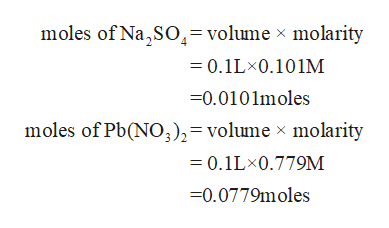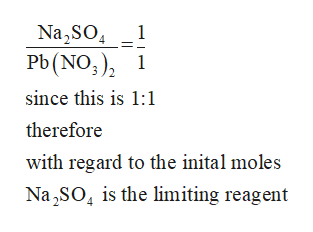# If 100.0 mL of 0.1010.101 M Na2SO4Na2SO4 is added to 100.0 mL of 0.7790.779 M Pb(NO3)2Pb(NO3)2, how many grams of PbSO4PbSO4 can be produced? Na2SO4(aq)+Pb(NO3)2(aq)⟶2NaNO3(aq)+PbSO4(s)Na2SO4(aq)+Pb(NO3)2(aq)⟶2NaNO3(aq)+PbSO4(s) STRATEGYCalculate the initial moles present and add them to a table of initial, change, and end of reaction moles.Determine the limiting reactant using the comparison-of-moles method.Use the limiting reactant to determine the change in moles for each reactant and product. Then, complete the table of moles.Convert the moles of PbSO4PbSO4 produced to the mass of PbSO4PbSO4, in grams.Step 1: Calculate the initial moles present and add them to the table. 0.101 mol Na2SO4L×1 L1000 mL× 100.0 mL=0.0101 mol Na2SO40.101 mol Na2SO4L×1 L1000 mL× 100.0 mL=0.0101 mol Na2SO4  0.779 molPb(NO3)2L×1 L1000 mL× 100.0 mL=0.0779 molPb(NO3)20.779 molPb(NO3)2L×1 L1000 mL× 100.0 mL=0.0779 molPb(NO3)2 molesNa2SO4(aq)Na2SO4(aq)++Pb(NO3)2(aq)Pb(NO3)2(aq)⟶⟶2NaNO3(aq)2NaNO3(aq)++PbSO4(s)PbSO4(s)initial0.01010.0101 0.07790.0779 0 0change? ? ? ?equilibrium? ? ? ?Step 2: Which reactant is limiting?Pb(NO3)2Pb(NO3)2Na2SO4

Question
3 views

If 100.0 mL of 0.1010.101 M Na2SO4Na2SO4 is added to 100.0 mL of 0.7790.779 M Pb(NO3)2Pb(NO3)2, how many grams of PbSO4PbSO4 can be produced?

Na2SO4(aq)+Pb(NO3)2(aq)⟶2NaNO3(aq)+PbSO4(s)Na2SO4(aq)+Pb(NO3)2(aq)⟶2NaNO3(aq)+PbSO4(s)

STRATEGY

1. Calculate the initial moles present and add them to a table of initial, change, and end of reaction moles.

2. Determine the limiting reactant using the comparison-of-moles method.

3. Use the limiting reactant to determine the change in moles for each reactant and product. Then, complete the table of moles.

4. Convert the moles of PbSO4PbSO4 produced to the mass of PbSO4PbSO4, in grams.

Step 1: Calculate the initial moles present and add them to the table.

0.101 mol Na2SO4L×1 L1000 mL× 100.0 mL=0.0101 mol Na2SO40.101 mol Na2SO4L×1 L1000 mL× 100.0 mL=0.0101 mol Na2SO4

0.779 molPb(NO3)2L×1 L1000 mL× 100.0 mL=0.0779 molPb(NO3)20.779 molPb(NO3)2L×1 L1000 mL× 100.0 mL=0.0779 molPb(NO3)2

moles Na2SO4(aq)Na2SO4(aq) ++ Pb(NO3)2(aq)Pb(NO3)2(aq) ⟶⟶ 2NaNO3(aq)2NaNO3(aq) ++ PbSO4(s)PbSO4(s)
initial 0.01010.0101   0.07790.0779   0   0
change ?   ?   ?   ?
equilibrium ?   ?   ?   ?
Step 2: Which reactant is limiting?
Pb(NO3)2Pb(NO3)2
Na2SO4
check_circle

Step 1

Given

Volume of the NaSO4 solution = 100mL

Molarity of the NaSO4    =0.101M

Volume of the Pb (NO3)2 solution= 100mL

Molarity of the Pb (NO3)2 = 0.779M

Step 2

Initial number of moleshelp_outlineImage Transcriptionclosemoles of Na,SO= volume x molarity 0.1LX0.101M =0.0101moles moles of Pb(NO),= volume x molarity =0.1LX0.779M 0.0779moles fullscreen
Step 3

According to the reaction the ra...help_outlineImage TranscriptioncloseNa2SO Pb(NO3)2 1 1 since this is 1:1 therefore with regard to the inital moles Na2SO is the limiting reagent fullscreen

### Want to see the full answer?

See Solution

#### Want to see this answer and more?

Solutions are written by subject experts who are available 24/7. Questions are typically answered within 1 hour.*

See Solution
*Response times may vary by subject and question.
Tagged in

### Quantitative analysis